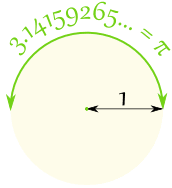is wrapped round the circle:1 Radian is about 57.2958 degrees.

Why "57.2958..." degrees? We will see in a moment.

The Radian is a pure measure based on the Radius of the circle:

and wrap it round the circle.

Let us see why 1 Radian is equal to 57.2958... degrees:In a half circle there are π radians, which is also 180°

=57.2958...°
(approximately)

To go from radians to degrees: multiply by 180, divide by π

To go from degrees to radians: multiply by π, divide by 180

Here is a table of equivalent values:

(exact)
(approx)
30° π/6 0.524
45° π/4 0.785
60° π/3 1.047
90° π/2 1.571
180° π 3.142
270° 3π/2 4.712
360° 2π 6.283### Example: How Many Radians in a Full Circle?

Imagine you cut pieces of string exactly the length from the center to the circumference of a circle ...

... how many pieces do you need to go once around the circle?

Because the radian is based on the pure idea of "the radius being laid along the circumference", it often gives simple and natural results when used in mathematics.

For example, look at the sine function for very small values:

 x sin(x) (radians) 1 0.1 0.01 0.001 0.841471 0.0998334 0.0099998 0.001

For very small values. "x" and "sin(x)" are almost the same
(as long as "x" is in Radians!)